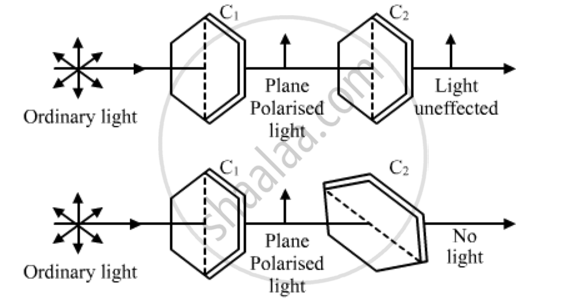# Using the Phenomenon of Polarisation, Show How the Transverse Nature of Light Can Be Demonstrated. - Physics

Using the phenomenon of polarisation, show how the transverse nature of light can be demonstrated.

#### Solution

Suppose that an ordinary light is incident normally on a pair of crystals C1 and C2. When the ordinary light passes through C1, it gets plane polarised in the direction perpendicular to the length of crystal. Now, we observe that when the axes of two crystals are parallel, the intensity of the emerging light will be maximum. When the second crystal is placed perpendicular w.r.t the first crystal, the intensity of light observed is zero. This is because the electric field of the plane polarised light obtained from C1 can vibrate only in one direction. Hence, when the axis of the crystal is perpendicular to its direction of vibration of electric field, it gets blocked. This depicts the transverse nature of light.Concept: Polarisation
Is there an error in this question or solution?